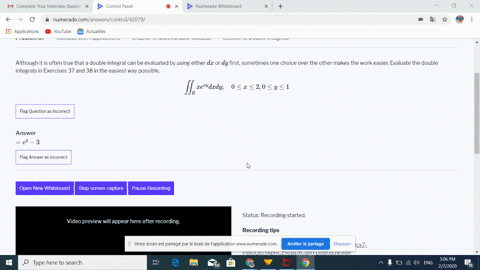Black Friday is Here! Start Your Numerade Subscription for 50% Off!Join Today### Although it is often true that a double integral …

07:54Georgia Southern University
Problem 36

# Find the volume under the given surface $z=f(x, y)$ and above the rectangle with the given boundaries.$$z=e^{x+y} ; 0 \leq x \leq 1.0 \leq y \leq 1$$

## Discussion

You must be signed in to discuss.

## Video Transcript

Okay, Another volume problem. So instead of our double integral x and Y are both going from zero to one in a grand A Z to the explosive all high So this is a supersymmetric and a girl I'Ll just put the eggs first of the matter Get on the wife first, Ok? And so anti derivative here. Well, it's just it's stuff. I mean, this is just about as easy as it gets. Justine, evaluate zero to one value extra zero one. So that's just okay. Me too. The one plus why minus c to the y. Okay. And then this is pretty straight forward again. Anti derivatives or just the exponential is themselves. You won. Okay, so if we evaluate at one will get he squared minus a and then minus evaluated zero. Me too. The one minus one. So what is that? He squared minus two e Plus, what